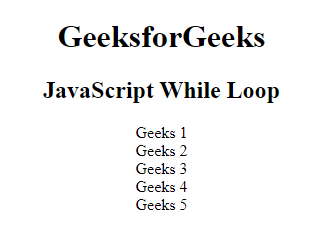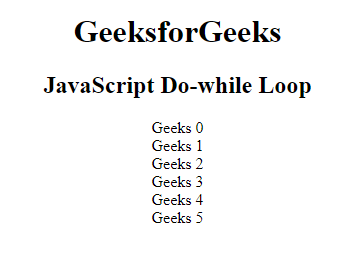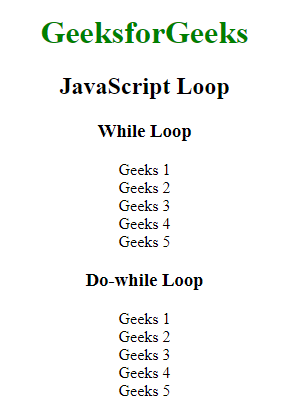# JavaScript While Loop

• Last Updated : 15 Nov, 2021

A While Loop in Javascript is a control flow statement that allows the code to be executed repeatedly based on the given boolean condition. The while loop can be thought of as a repeating if statement.

The loop can be used to execute the specific block of code multiple times until it failed to match the condition.

There are mainly two types of loops:

• Entry Controlled loops: In this type of loop, the test condition is tested before entering the loop body. For Loop and While Loop are entry-controlled loops.
• Exit Controlled Loops: In this type of loop the test condition is tested or evaluated at the end of the loop body. Therefore, the loop body will execute at least once, irrespective of whether the test condition is true or false. the do-while loop is exit controlled loop.

Syntax:

```while (condition) {
// Statements
}```

Example: This example illustrates the use of a while loop.

## HTML

 `` `<``html``>`   `<``head``>` `    ``<``title``>JavaScript While loop` ``   `<``body` `style``=``"text-align:center;"``>` `    ``<``div``>` `        ``<``h1``>GeeksForGeeks` `        ``<``h2``>JavaScript While Loop` `    ``` `    ``<``p` `id``=``"GFG"``>`   `    `  `    ``` `    ``<``script``>` `    ``var print = "";` `    ``var val = 1;` `    ``while(val < ``6``) {` `        ``print += "Geeks " + val;` `        ``print += "
"` `        ``val += 1;` `    ``}` `    ``document.getElementById("GFG").innerHTML = print;` `    ``` ``   ``

Output:while loop

Do-While loop: A do-while loop is a control flow statement that executes a block of code at least once, and then repeatedly executes the block or not depending on a given boolean condition at the end of the block.

Syntax:

```do {
// Statements
}
while (condition);```

Example: This example illustrates the use of the do-while loop.

## HTML

 `` `<``html``>`   `<``head``>` `    ``<``title``>JavaScript While loop` ``   `<``body` `style``=``"text-align:center;"``>` `    ``<``div``>` `        ``<``h1``>GeeksforGeeks` `        ``<``h2``>JavaScript Do-while Loop ` `    ``<``p` `id``=``"GFG"``>`   `    `  `    ``` `    ``<``script``>` `    ``var print = ""` `    ``var val = 0;` `    ``do {` `        ``print += "Geeks " + val;` `        ``print += "<``br``>";` `        ``val += 1;` `    ``}` `    ``while (val < ``6``);` `    ``document.getElementById("GFG")``.innerHTML` `= ``print``;` `    ``` ``   ``

Output:Do-while Loop

Comparison between while and do-while loop: The do-while loop executes the content of the loop once before checking the condition of the while loop. While the while loop will check the condition first before executing the content.

Example: This example illustrates both while and do-while loops.

## HTML

 `` `<``html``>`   `<``head``>` `    ``<``title``>JavaScript loop` ``   `<``body` `style``=``"text-align:center;"``>` `    ``<``div``>` `        ``<``h1` `style``=``"color:green;"``>` `            ``GeeksforGeeks` `        ``` `        ``<``h2``>JavaScript Loop ` `    ``<``h3``>While Loop` `    ``<``p` `id``=``"GFG"``>`   `    `  `    ``` `    ``<``script``>` `    ``var text = "";` `    ``var i = 1;` `    ``while(i < ``6``) {` `        ``text += "Geeks " + i + "
";` `        ``i++;` `    ``}` `    ``document.getElementById("GFG").innerHTML = text;` `    ``` `    ``<``h3``>Do While Loop` `    ``<``p` `id``=``"GFG1"``>`   `    ``` `    ``<``script``>` `    ``var text = ""` `    ``var i = 1;` `    ``do {` `        ``text += "Geeks " + i + "<``br``>";` `        ``i++;` `    ``}` `    ``while (i < ``6``);` `    ``document.getElementById("GFG1")``.innerHTML` `= ``text``;` `    ``` ``   ``

Output:Javascript Loop

My Personal Notes arrow_drop_up
Recommended Articles
Page :